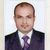# QlikView App Dev

Discussion Board for collaboration related to QlikView App Development.

Announcements
On May 18th at 10AM EDT we will answer your QlikView questions live. REGISTER
cancel
Showing results for
Did you mean:Not applicable

## Calculate the total spend of top N vendors, by vendor category

I'm currently working on a problem that has been taxing me for a while.

I am trying to produce a stacked bar chart showing the % spend of the top 10 vendors, % spend of the next 10 vendors and the remaining spend, by vendor category.

I can calculate the spend of the top N vendors but I have to include the vendor as a dimension in the chart, which defeats the purpose of what I am doing.

• Top 10 =IF(aggr(rank(sum([NET_Amount])),vndProclass_Lvl1,vndVendor_Name)<11,Sum([NET_Amount]))

• Next 10 =(IF(aggr(rank(sum([NET_Amount])),vndProclass_Lvl1,vndVendor_Name)>10,IF(aggr(rank(sum([NET_Amount])),vndProclass_Lvl1,vndVendor_Name)<=20,Sum([NET_Amount]))))

• Remaining = IF(aggr(rank(sum([NET_Amount])),vndProclass_Lvl1,vndVendor_Name)>20,Sum([NET_Amount]))

What I actually want is to have category as the only dimension and then three expressions, one for each bar segment but my current expressions rely on vndVendor_Name being a dimension.

E.g. Category | Total spend for top 10 suppliers | Total spend for next 10 suppliers | Remaining spend

Can anyone help me with this? It's really beginning to bug me.

1 Solution

Accepted SolutionsPartner

hei attach is an example

the logic is ranking the vendor to group in calculated dimension

hope it helps you

4 RepliesPartner

hei attach is an example

the logic is ranking the vendor to group in calculated dimension

hope it helps youMVP

Hi,

Use Range sum function in place of Rank function.

Rgds

AnandNot applicable
Author

Great! Thank you for your help!

I hadn't thought of the problem the same way but that has been very helpful.

Alex B.Not applicable
Author

Hi Liron,

I have managed to use your suggestion and it works great, thanks. However I was hoping to show each segment of the stacked bar as a % of the total for that category. This means they should all add up to 100.

I thought I could just do the following within the expression in order to calculate each section as a percentage of the total for the category but it doesn't do what I anticipated.

= sum(NET_Amount) / Aggr(sum(NET_Amount),Category)

Any suggestions?